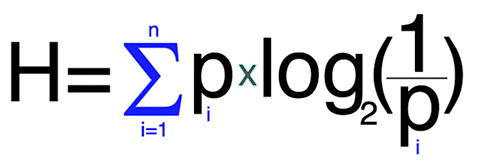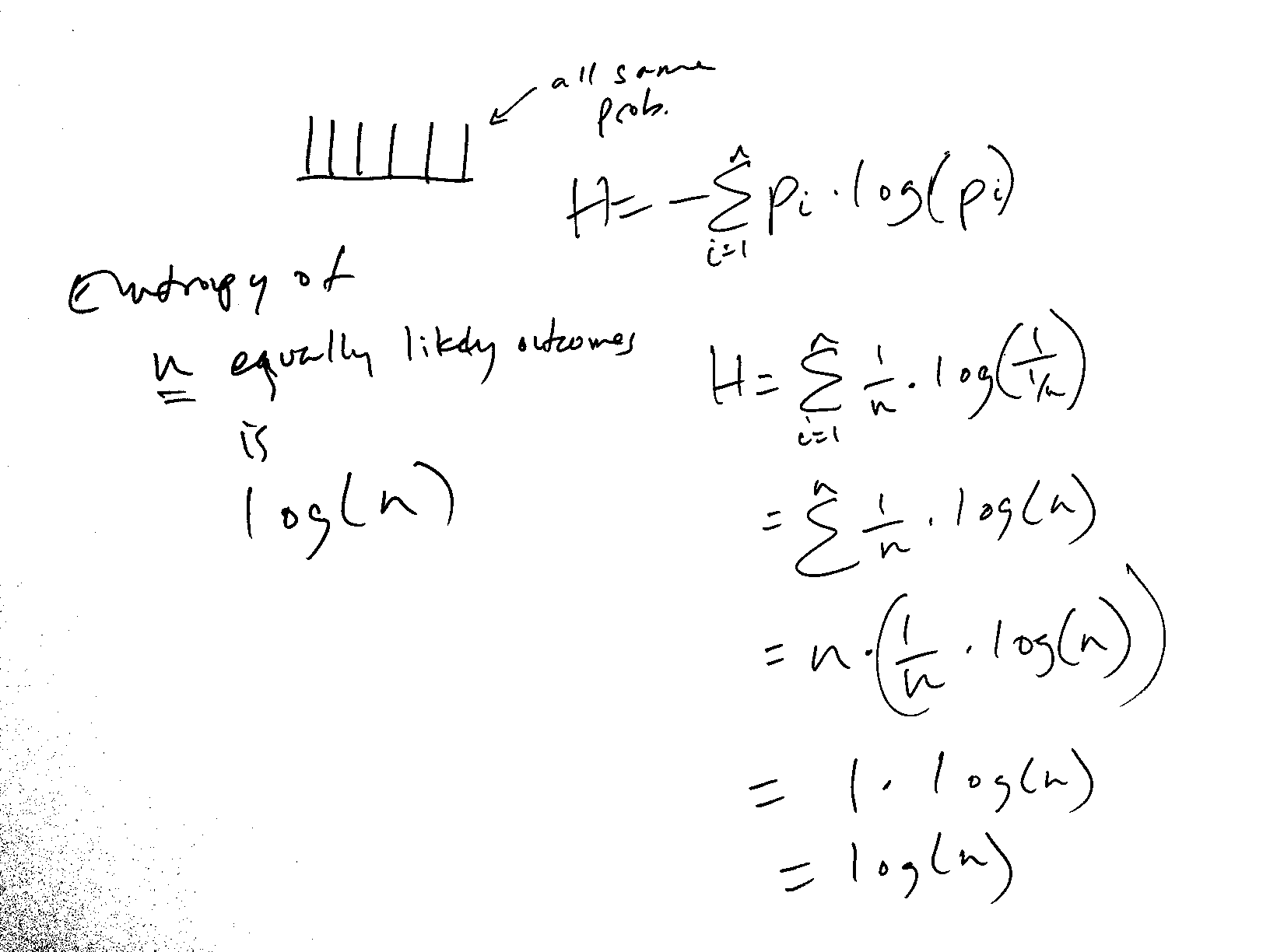I've heard the term "entropy" over the years and never understood it until now. I used to think entropy was randomness, and it's kind of related.

Entropy can be defined in a few ways, some more complicated than others.

Entropy is a measure of how much information is gained (measured in bits) when an outcome is revealed.

Let's say you have a coin flip with a fair coin. There is probability of 1/2 that it will be heads, and 1/2 that it will be tails. Since either outcome is equally likely, entropy, or the unpredictability of the outcome, is at its maximum.

If you had an unfair coin, and knew that 60% of the time it would be heads, then entropy has decreased, because there is some predictability in play.

How much has the entropy decreased?

Shannon's equation of measuring entropy, here represented as H, is the sum of the probabilities of each of the outcomes time the logarithm of the 1/probability of each outcome.We simplify 1/probability as probability-1, and moving the exponent out of the logarithm as -1, and multiplying it by the whole thing. This simplifies the log portion to log2(p). I use the form in the image in the calculations below.

Fair coin: H = 2 * (.5 * log2(1/(.5))) = 1
Unfair coin: H = 2 * (.6 * log2(1/(.6))) = 0.736966
2-headed coin: H = 2 * (1 * log2(1/1)) = 0

So the entropy decreased by 0.27 bits of information. When entropy decreases from its maximum, that means that information has leaked. This information leak can be exploited in naive versions of cryptography to break it. Modern cryptography maximizes entropy so that the encryption output is indistinguishable from randomness.

Note the 2-headed coin has 0 entropy, because the outcome is reliably predictable.

## My Simplification

I went to the board and figured out that n equally likely outcomes have the entropy of log2(n). So entropy for a coin flip or a dice roll are different. A higher n means a higher entropy because the unpredictability increases as n increases.Coin flip (fair coin): 1
Dice roll (fair die, 6-sized): 2.58
Dice roll (fair die, 10-sized): 3.32
Dice roll (fair die, 20-sized): 4.32

For powers of 2, these are easy to calculate, because log28 = 3.

Shannon conjectured that the minimum encoding for n bits was log2n. It takes 1 bit to encode 2 items of information, 2 bits to encode 4 (00, 01, 10, 11). 3 bits to encode 8, 5 bits to encode 32. In encoding integers in binary, since we add 0 into the mix for integers, it takes log2n bits to encode integers from 0 to n - 1.

## What's It Good For?

Entropy comes into play in:

• compression
• encryption
• data transmission
• error detection and correction

So it's worthwhile to learn!